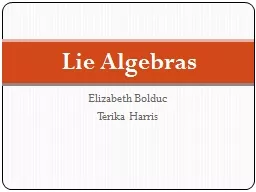# Elizabeth Bolduc Terika Harris - PowerPoint Presentation#### Elizabeth Bolduc Terika Harris - Description

Lie Algebras Lie Algebras Definition Let be a vector space over some field and let and is a Lie Algebra with a binary operation known as the Lie Bracket if the following are true ID: 702387 Download Presentation

### Tags

algebra lie identity algebras lie algebra algebras identity alternating bilinear bracket space field groups definition vector group anticommutative implies

Embed / Share - Elizabeth Bolduc Terika Harris

## Presentation on theme: "Elizabeth Bolduc Terika Harris"— Presentation transcript

Slide1

Elizabeth BolducTerika Harris

Lie AlgebrasSlide2

Lie Algebras: Definition

Let

be a vector space over some field,

and let and . is a Lie Algebra with a binary operation, known as the Lie Bracket if the following are true:BilinearAlternatingJacobi identity Note: Bilinear and Alternating implies anticommutative.

Slide3

A Misconception

Marius Sophus

Lie

Norwegian Mathematician Geometry and differential equationsSlide4

Lie Groups

D

ifferentiable manifold

Such that the operations are compatible with the smooth structure. ExamplesSlide5

How do Lie Algebras Relate to Lie Groups?

Lie Algebras help us understand Lie Groups.

If G is a Lie Group, the Lie Algebra of G is defined as the tangent space of the identity element of G. Slide6

Example:

Slide7

Hermann Weyl

Introduced in 1930

Character Formula

SymmetrySlide8

Lie Algebras: Definition

Let

be a vector space over some field,

and let and . is a Lie Algebra with a binary operation, known as the Lie Bracket if the following are true:BilinearAlternatingJacobi identity Note: Bilinear and Alternating implies anticommutative.

Slide9

Example

Consider any associative algebra,

over some field

.Now we can define our Lie Bracket to be Slide10

Check that this is a Lie Algebra

Let a, b

Bilinear Alternating Jacobi Identity

+

Slide11

Example

General Linear Group:

Slide12

Example: Cross Product

with multiplication defined by the cross product

Bilinear?

Alternating?Jacob Identity? Slide13

Example

Abelian

Lie Algebra

If every bracket product is zeroQuestion: can you name this Abelian Lie Algebra?Slide14

Lie Algebras: Definition

Let

be a vector space over some field,

and let and . is a Lie Algebra with a binary operation, known as the Lie Bracket if the following are true:BilinearAlternatingJacobi identity Note: Bilinear and Alternating implies anticommutative.

Slide15

Conclusion

Every Lie Group has a corresponding Lie Algebra.

Lie Algebras help us understand Lie Groups

Shom More....By: faustina-dinatale
Views: 2
Type: Public